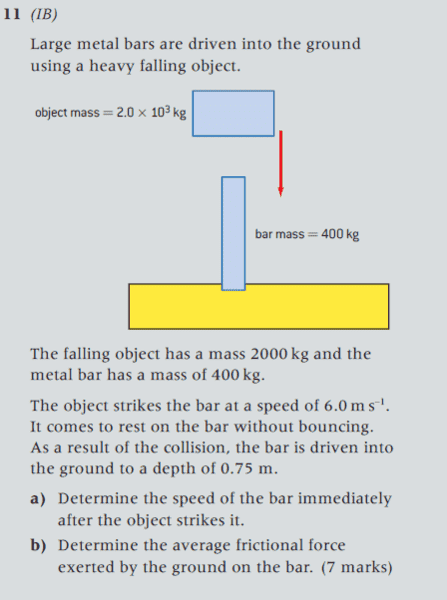# Law Conservation of Energy: Starting off a solution for 11b

## Homework Statement## Homework Equations

ΔEsystem + ΔEsurroundings =0

## The Attempt at a Solution

I have solved 11a and got 5m/s.
I can do 11b just by just jumping to Ek = Fs (from the work kinetic energy theorem), but I would like to do it formally from first principles using the Law of Conservation of Energy.
I would then like to make use of
ΔEsystem + ΔEsurroundings =0
and then make use of ΔEsystem = ΔU + ΔEk + ΔEp
and then make use of ΔU = W + Q
and then introduce W = Fs
then solve for F.
However I am not sure where to put the system boundary so this is solvable. Then the question of the pluses and minuses.

Many thanks

#### Attachments

Last edited by a moderator:

•